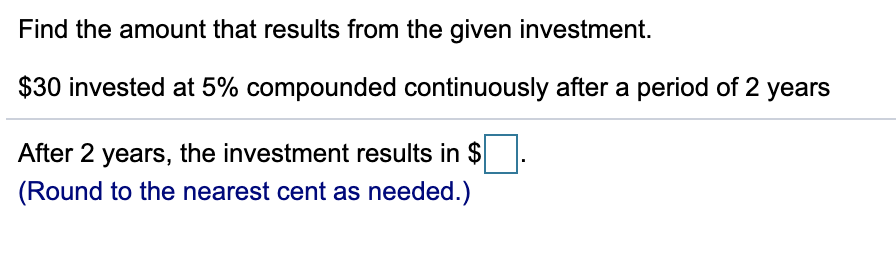# Find the amount that results from the given investment.\$30 invested at 5% compounded continuously after a period of 2 yearsAfter 2 years, the investment results in \$(Round to the nearest cent as needed.)

Question
1 viewshelp_outlineImage TranscriptioncloseFind the amount that results from the given investment. \$30 invested at 5% compounded continuously after a period of 2 years After 2 years, the investment results in \$ (Round to the nearest cent as needed.) fullscreen
check_circle

Step 1

From the given information it is required to calculate the amount that results from the given investment:

Principal (P) = \$30

Rate (r) = 5% = 5/100 = 0.05

Time (t) = 2 years

The formula to calculate the amount that is compounded continuously is:

Step 2

Now, put in the values to c...

### Want to see the full answer?

See Solution

#### Want to see this answer and more?

Solutions are written by subject experts who are available 24/7. Questions are typically answered within 1 hour.*

See Solution
*Response times may vary by subject and question.
Tagged in

### Algebra